Skip to main content Accessibility help
Home
Hostname: page-component-77ffc5d9c7-kttml Total loading time: 0.209 Render date: 2021-04-23T15:29:37.801Z Has data issue: true Feature Flags: { "shouldUseShareProductTool": true, "shouldUseHypothesis": true, "isUnsiloEnabled": true, "metricsAbstractViews": false, "figures": false, "newCiteModal": false, "newCitedByModal": true }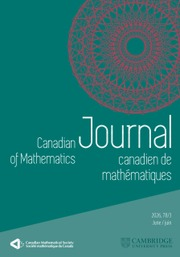Canadian Journal of Mathematics

# Fubini's Theorem for Ultraproducts of Noncommutative Lp -Spaces

Published online by Cambridge University Press:  20 November 2018

Corresponding
E-mail address:

## Abstract

Let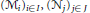${{({{\mathcal{M}}_{i}})}_{i}}{{_{\in }}_{I}},{{({{\mathcal{N}}_{j}})}_{j\in J}}$ be families of von Neumann algebras and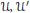$\mathcal{U},\,{\mathcal{U}}'$ be ultrafilters in$I$ ,$J$ , respectively. Let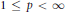$1\,\le \,p\,<\,\infty$ and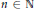$n\,\in \,\mathbb{N}$ . Let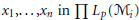${{x}_{1}},...,{{x}_{n}}\,\text{in}\,\prod {{L}_{p}}({{\mathcal{M}}_{i}})$ and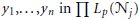${{y}_{1}},...,{{y}_{n\,}}\text{in }\Pi \,{{L}_{p}}({{\mathcal{N}}_{j}})$ be bounded families. We show the following equality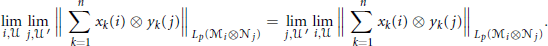$$\underset{i,\mathcal{U}}{\mathop{\lim }}\,\,\underset{j,{\mathcal{U}}'}{\mathop{\lim }}\,\,{{\left\| \sum\limits_{k=1}^{n}{{{x}_{k}}(i)\,\otimes \,{{y}_{k}}(j)} \right\|}_{{{L}_{p}}({{\mathcal{M}}_{i}}\otimes {{\mathcal{N}}_{j}})}}=\,\underset{j,{\mathcal{U}}'}{\mathop{\lim }}\,\,\underset{i,\mathcal{U}}{\mathop{\lim }}\,\,{{\left\| \sum\limits_{k=1}^{n}{{{x}_{k}}(i)\,\,\otimes \,{{y}_{k}}(j)} \right\|}_{{{L}_{p}}({{\mathcal{M}}_{i}}\otimes {{\mathcal{N}}_{j}})}}$$

For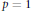$p\,=\,1$ this Fubini type result is related to the local reflexivity of duals of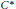${{C}^{*}}$ -algebras. This fails for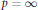$P=\infty$ .

## Keywords

Type
Research Article
Information
Canadian Journal of Mathematics , 01 October 2004 , pp. 983 - 1021
Copyright
Copyright © Canadian Mathematical Society 2004

## References

[BL] Bergh, J. and Löfström, J., Interpolation spaces. An introduction; Grundlehren der Mathematischen Wissenschaften 223, Springer-Verlag, Berlin, 1976.CrossRefGoogle Scholar
[BP] Blecher, D. P. and Paulsen, V. I., Tensor products of operator spaces. J. Funct. Anal. 99(1991), 262292.CrossRefGoogle Scholar
[Ch] Choi, M. D., A Schwarz inequality for positive linear maps on C*-algebras, Illinois J. Math 18, 565574.CrossRefGoogle Scholar
[C1] Connes, A., Classification of injective factors. Cases II 1, II , III λ , λ ≠ 1 . Ann. of Math. 104(1976), 73115.CrossRefGoogle Scholar
[C2] Connes, A., Sur la théorie non commutative de l’integration; Algèbres d’opérateurs, Semin. Les Plans-sur-Bex 1978, Lect. Notes Math. 725, 19143 (1979).Google Scholar
[C3] Connes, A., Noncommutative Geometry Academic Press, 1994.Google Scholar
[Dx] Dixmier, J., Von Neumann Algebras. North Holland, Amsterdam, 1981.Google Scholar
[EJR] Effros, E., Junge, M. and Ruan, Z. J., Integral mappings and the principle of local refelxivity for noncommutative L 1 -spaces. Annals of Math. 151(2000), 5992.CrossRefGoogle Scholar
[ER2] Effros, E. and Ruan, Z-J., On approximation properties of operator spaces. Internat. J. Math. 1(1990), 163187.CrossRefGoogle Scholar
[ER3] Effros, E. and Ruan, Z-J., Operator spaces, London Math. Soc. Monographs New Series 23, Oxford University Press, 2000.Google Scholar
[Fi] Fidaleo, F., Canonical operator space structures on non-commutative Lp spaces. J. Funct. Anal. 169(1999), 226250.CrossRefGoogle Scholar
[Gr] Groh, U., Uniform ergodic theorems for identity preserving Schwartz maps on W*-algebras. J. Operator Theory 11(1984), 396404.Google Scholar
[Ha1] Haagerup, U., A new proof of the equivalence of injectivity and hyperfiniteness for factors on a separable Hilbert space. J. Funct. Anal. 62(1985), 160201.CrossRefGoogle Scholar
[Ha2] Haagerup, U., Lp-spaces associated with an arbitrary von Neumann algebra. Algébres d’opérateurs et leurs applications en physique mathématique (Proc. Colloq., Marseille, 1977), pp. 175184, Colloq. Internat. CNRS, 274, CNRS, Paris, 1979.Google Scholar
[J1] Junge, M., Doob's inequality for non-commutative martingales. J. Reine Angew. Math 549(2002), 149190.Google Scholar
[J2] Junge, M., Applications of the Fubini theorem for non-commutative Lp spaces; preprint.Google Scholar
[JNRX] Junge, M., Nielsen, N. J., Ruan, Z. J. and Xu, Q., The local structure of non-commutative Lp spaces. to appear in Advances of Mathematics.Google Scholar
[JR] Junge, M. and Ruan, Z. J., Approximation properties for non-commutative Lp spaces associated to discrete groups. Duke Math. J. 117(2003), 313341.CrossRefGoogle Scholar
[JX] Junge, M. and Xu, Q.. Non-commutative Burkholder/Rosenthal inequalities. Annals of Probability 31(2003), 948995.Google Scholar
[Ki] Kirchberg, E., On nonsemisplit extensions, tensor products and exactness of group C*-algebras. Invent. Math. 112(1993), no. 3,CrossRefGoogle Scholar
[Ko] Kosaki, H., Applications of the Complex Interpolation Method to a von Neumann Algebra: Non-commutative Lp-spaces. J. Funct. Anal. 56(1984), 2978.CrossRefGoogle Scholar
[Ko2] Kosaki, H., On the continuity of the map φ → |φ| from the predual of aW*-algebra. J. Funct. Anal. 59(1984), 123131.CrossRefGoogle Scholar
[KR] Kadison, R. and Ringrose, J., Fundamentals of the theory of operator algebras I and II. Graduate Studies in Mathematics 15 and 16, AMS, Providence, RI, 1997 Google Scholar
[Ne] Nelson, E., Notes on non-commutative integration. J. Funct. Anal. 15(1974), 103116.CrossRefGoogle Scholar
[Pa] Paulsen, V. I., Completely bounded maps and dilations. Pitman Research Notes in Mathematics, 1986.Google Scholar
[PT ] Pedersen, G. and Takesaki, M., The Radon-Nikodym theorem for von Neumann algebras. Acta Math. 130(1973), 5387.CrossRefGoogle Scholar
[P6] Pisier, G., The operator Hilbert space OH, complex interpolation and tensor norms. Memoirs Amer. Math. Soc. 585, Vol 122, July 1996.CrossRefGoogle Scholar
[P7] Pisier, G., Noncommutative vector valued integration and completely p-summing maps. Astérisque No. 247, (1998).Google Scholar
[P8] Pisier, G., Introduction to operator spaces; preprint.Google Scholar
[R1] Ruan, Z. J., Subspaces of C*-algebras. J. Funct. Anal. (1988), 217230.CrossRefGoogle Scholar
[Ra2] Raynaud, Y., On ultrapowers of non-commutative Lp-spaces. J. Operator Theory 48(2002), 4168.Google Scholar
[Se] Segal, I. E., A non-commutative extension of abstract integration. Ann. Math. 57(1953), 401457.CrossRefGoogle Scholar
[Te1] Terp, M., Lp-spaces associated with von Neumann algebras I and II; Copenhagen Univ. 1981.Google Scholar
[Tk] Takesaki, M., Theory of operator algebras I. Springer-Verlag, New York, 1979.CrossRefGoogle Scholar
[Wa] Wassermann, S.. On tensor products of certain group C*-algebras. J. Funct. Analysis 23(1976), 239254.CrossRefGoogle Scholar

### Full text views

Full text views reflects PDF downloads, PDFs sent to Google Drive, Dropbox and Kindle and HTML full text views.

Total number of HTML views: 0
Total number of PDF views: 61 *
View data table for this chart

* Views captured on Cambridge Core between 20th November 2018 - 23rd April 2021. This data will be updated every 24 hours.You have Access

# Send article to Kindle

To send this article to your Kindle, first ensure no-reply@cambridge.org is added to your Approved Personal Document E-mail List under your Personal Document Settings on the Manage Your Content and Devices page of your Amazon account. Then enter the ‘name’ part of your Kindle email address below. Find out more about sending to your Kindle. Find out more about sending to your Kindle.

Note you can select to send to either the @free.kindle.com or @kindle.com variations. ‘@free.kindle.com’ emails are free but can only be sent to your device when it is connected to wi-fi. ‘@kindle.com’ emails can be delivered even when you are not connected to wi-fi, but note that service fees apply.

Find out more about the Kindle Personal Document Service.

Fubini's Theorem for Ultraproducts of Noncommutative Lp -Spaces
Available formats
×

# Send article to Dropbox

To send this article to your Dropbox account, please select one or more formats and confirm that you agree to abide by our usage policies. If this is the first time you use this feature, you will be asked to authorise Cambridge Core to connect with your <service> account. Find out more about sending content to Dropbox.

Fubini's Theorem for Ultraproducts of Noncommutative Lp -Spaces
Available formats
×

# Send article to Google Drive

To send this article to your Google Drive account, please select one or more formats and confirm that you agree to abide by our usage policies. If this is the first time you use this feature, you will be asked to authorise Cambridge Core to connect with your <service> account. Find out more about sending content to Google Drive.

Fubini's Theorem for Ultraproducts of Noncommutative Lp -Spaces
Available formats
×
×

#### Conflicting interests

Do you have any conflicting interests? *